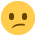# Sandy you'll know this!

On the June 09 MAC paper Task 2.2 a iv) It asks you to calculate the direct labour cost per hr.

I divided the Direct Labour Cost of £3,600,000 by Labours Hours worked which was 180. This gave me the answer of £20,000. But the answer is £20. HuhI thought it might be I have put too many zeros but on the question before it asks you to calculate materials cost as a % of turnover. I did this as £6,750,000/£22,500,000 x 100 = 30%. This is correct according to the answers!

So should there be three zeros after the given figures or not?